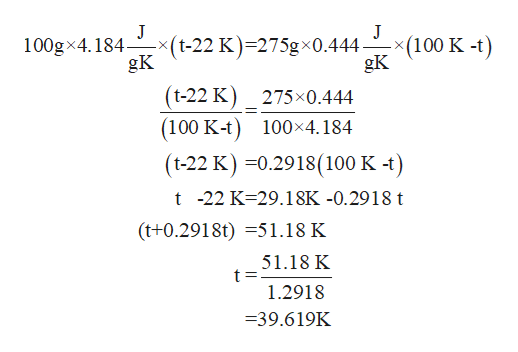# (100g)(4.184J/g K)(t-22)=(275g)(0.444J/g K)(t-100)What is the step by step solution to this problem?

Question

(100g)(4.184J/g K)(t-22)=(275g)(0.444J/g K)(t-100)

What is the step by step solution to this problem?

check_circleExpert Solution
Step 1

The given equation is

Step 2

The only unknown in the equation is‘t’.

This the equations of heat transfer betwee...help_outlineImage TranscriptioncloseJ -x(t-22 K)=275gx0.444 gK J (100 K -t) gK 100gx4.184- (t-22 K) 275x0.444 (100 K-t) 100x4.184 (t-22 K) 0.2918(100 K -t) t -22 K 29.18K -0.2918 t (t+0.2918t)51.18 K 51.18 K 1.2918 -39.619K fullscreen

### Want to see the full answer?

See Solution

#### Want to see this answer and more?

Solutions are written by subject experts who are available 24/7. Questions are typically answered within 1 hour*

See Solution
*Response times may vary by subject and question
Tagged in

### Chemistry